output.to from Sideway
Calculus

Applications of Integration

Bounded Area under Curve

Draft for Information Only

# Content

``` Bounded Area of a Function  Bounded Area of a Curve which is entirely above the x-axis    Bounded Area of a Curve which is entirely below the x-axis    Bounded Area of a Curve which is partly above and partly below the x-axis     Net Bounded Area of a function:   Total Bounded Area of a function:  Bounded Area of a Curve through transformation   Geometrical Representation of Integration by Parts:```

# Bounded Area of a Function

Definite integral can be interpreted as the net signed area of the curve bounded by the curve of function f(x) and the x-axis on the closed interval [a,b].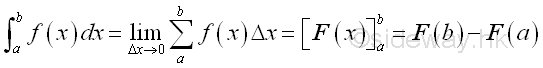Since Δx is always positive, each infinitesimal element of area will have the same sign as the value of function f(x). The infinitesimal element of area can be positive, negative or zero. The infinitesimal element of area is called the signed area under the curve because the positive area is under the curve and the negative area is above the curve. Therefore the summation of all infinitesimal elements is the net signed bounded area.  And for problems of calculating the total bounded area of a function or concerning with the sign of the infinitesimal elements, the shape of the curve should be determined before applying the method of integration.

## Bounded Area of a Curve which is entirely above the x-axis

When the curve of a function f(x) is entirely above the x-axis, the area obtained by integration is positive and all infinitesimal elements are under the curve.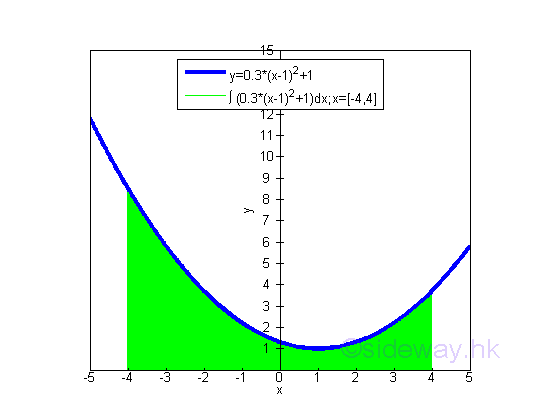The bounded area underneath the curve of function y=0.3(x-1)2+1 between the closed interval -4 and 4 and above the x-axis is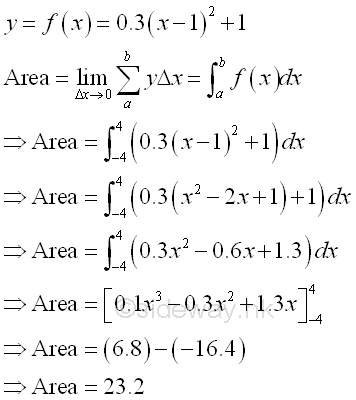The bounded area under the curve and  above the x-axis is positive and is equal to 23.2. But the integral of a function can be positive, negative or zero.

## Bounded Area of a Curve which is entirely below the x-axis

When the curve of a function f(x) is entirely below the x-axis, the area obtained by integration is negative and all infinitesimal elements are above the curve.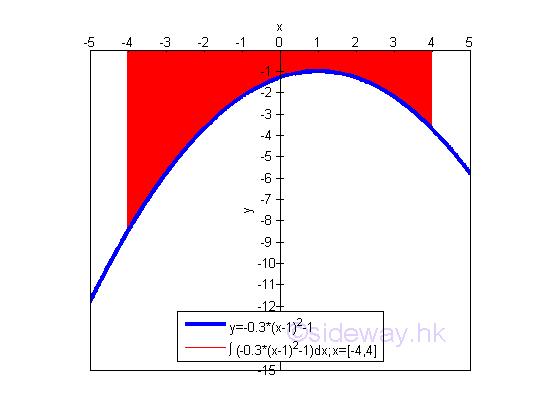The bounded area above the curve of function y=-0.3(x-1)2-1 between the closed interval -4 and 4 and below x-axis is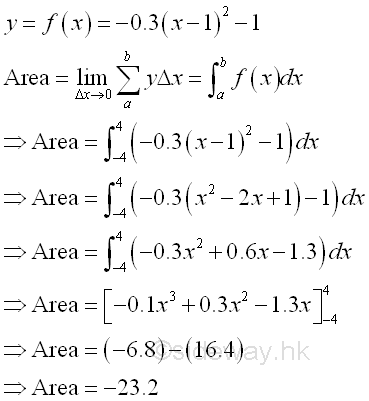The bounded area above the curve and below the x-axis is negative and is equal to -23.2. The area  is the absolute value of the signed area. But the integral of a function can be positive, negative or zero.

## Bounded Area of a Curve which is partly above and partly below the x-axis

When the curve of a function f(x) is partly above and partly below the x-axis, the area obtained by integration is the net signed bounded area because the infinitesimal elements can be under or above the curve.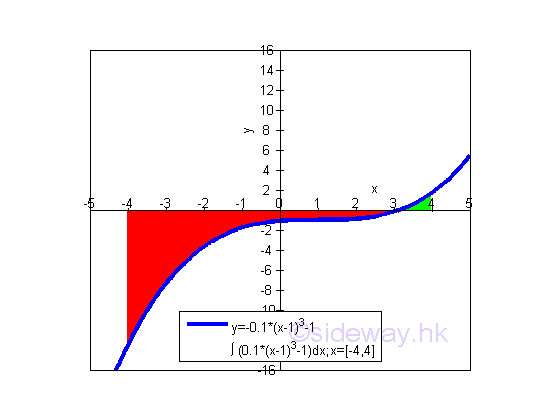### Net Bounded Area of a function:

The net bounded area under the curve of function y=0.1(x-1)3-1 between the closed interval -4 and 4  of x axis is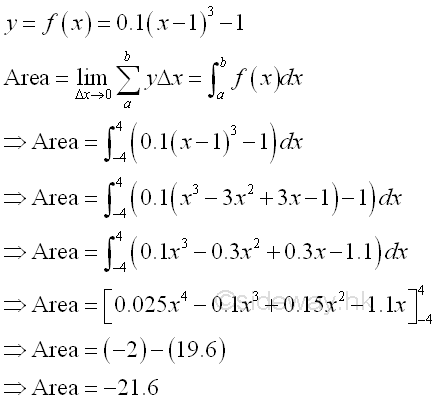The net bounded area bounded by the curve and the x-axis is negative and is equal to -21.6. The area  is the absolute value of the signed area. But the integral of a function can be positive, negative or zero.

### Total Bounded Area of a function:

In order to calculate the total bounded area, the shape of the function f(x) should be determined first. The x-intersect of f(x) when y=0 is equal to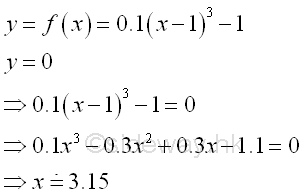The bounded area bounded by the curve and below the x-axis is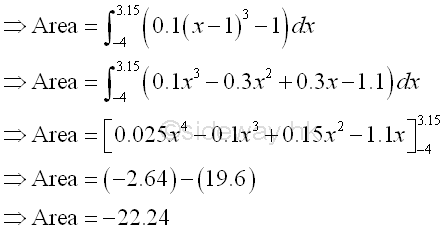The bounded area bounded by the curve and above the x-axis is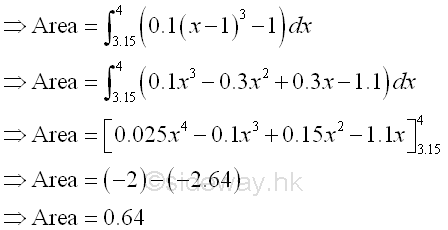Therefore the net bounded area bounded by the curve is -22.24+0.64=-21.6 as before and the total bounded area bounded by the curve is the sum of absolute area, i.e. 22.24+0.64=22.88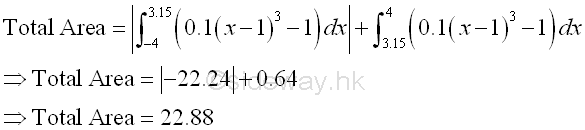## Bounded Area of a Curve through transformation

Since finding the bounded area involving the finding of integral, sometimes finding the bounded area of a curve is much easier to sum vertically than to sum horizontally, that is using an easier way of finding the integral.

And mathematically, the relationship between the bounded area by horizontal summation and the bounded area by vertical summation is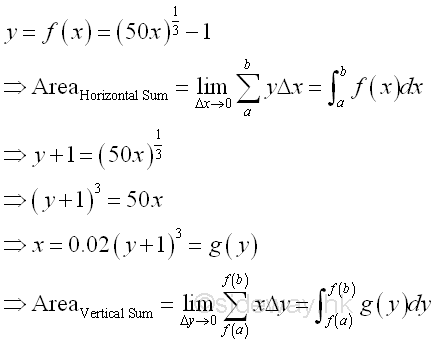There is only a direct relationship between function f(x) and function g(x). And there is no direct relatonship between the horizontally summed area and the vertically summed area. In order to simplify the task of finding the integral, a transformation of the bounded area calculation is needed. The bounded area transformation can be carried out by integration by parts through the transformation of variable of integration.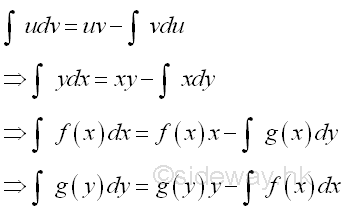### Geometrical Representation of Integration by Parts:

Through variable of integration transformation, the formula of integration by parts can be used for the transformation of bounded area summation.

In the formulas of integration by parts above, the x is obtained from dx through integration or the y is obtained from dy through integration. The practical forms of integration by parts are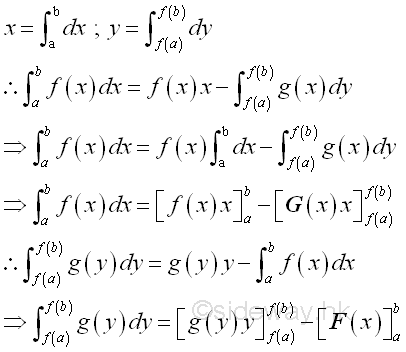The first integral on the right hand side of the two integration by parts formulas above are equal because they are both derived from xy,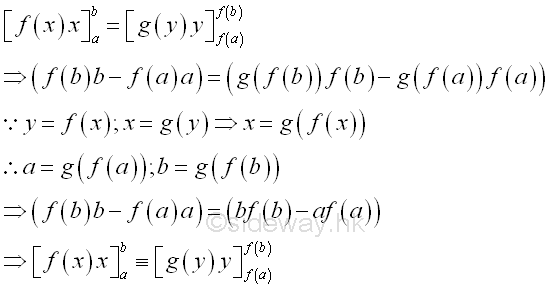Therefore the term xy in the formula of integration by parts is equal to the product of the upper limits of x and y minus the product of the lower limits of x and y.

Geometrically, the relationship between the bounded area by horizontal summation and the bounded area by vertical summation is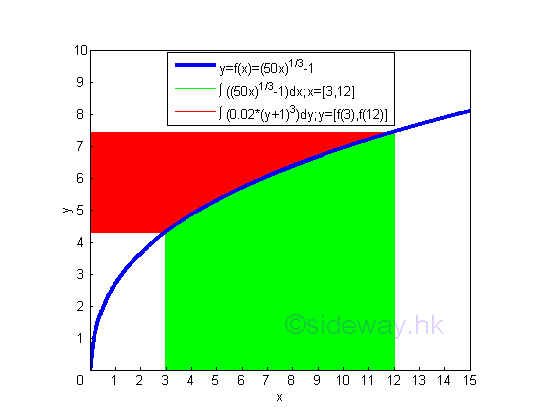According to the graph, the sum of bounded red area and bounded green area is equal to the rectangular area formed by the upper limits (12,f(12)) and the origin (0,0) minus the rectangular area formed by the lower limits (3,f(3)) and the origin (0,0).

The bounded green area by horizontal summation is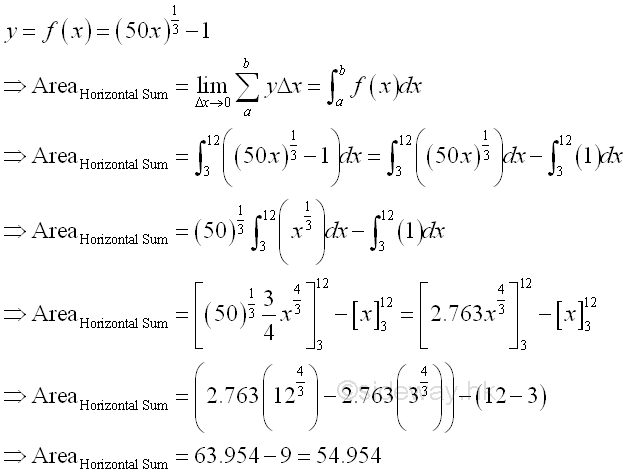The bounded red area by vertical summation is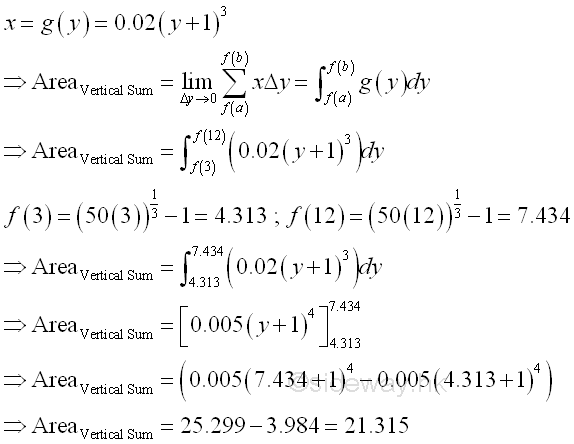And the area formed by the two rectangular areas is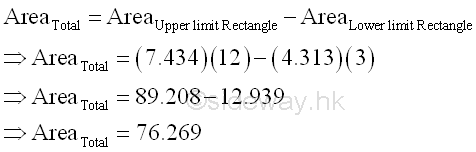Therefore the term xy in the formula of integration by parts can be considered as the net total area of the net area of vertical sum plus the net area of horizontal sum, imply.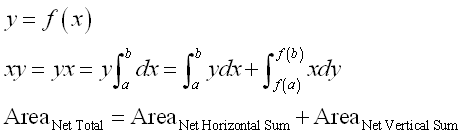©sidewayReferences

1. S. James, 1999, Calculus, Brooks/Cole Publishing Co., USA
2. B. Joseph, 1978, University Mathematics: A Textbook for Students of Science & Engineering, Blackie & Son Limited, HongKongID: 111100004 Last Updated: 11/21/2011 Revision: 0 Ref:Home (5)

Business

Management

HBR (3)

Information

Recreation

Hobbies (7)

Culture

Chinese (1097)

English (336)

Reference (66)

Computer

Hardware (149)

Software

Application (187)

Digitization (24)

Numeric (19)

Programming

Web (644)CSS (SC)

ASP.NET (SC)

HTML

Knowledge Base

Common Color (SC)

Html 401 Special (SC)

OS (389)MS Windows

Windows10 (SC)

.NET Framework (SC)

DeskTop (7)

Knowledge

Mathematics

Formulas (8)

Number Theory (206)

Algebra (20)

Trigonometry (18)

Geometry (18)

Calculus (67)

Complex Analysis (21)

Engineering

Tables (8)

Mechanical

Mechanics (1)

Rigid Bodies

Statics (92)

Dynamics (37)

Fluid (5)

Control

Acoustics (19)

Biology (1)

Geography (1)

Latest Updated Links

Copyright © 2000-2019 Sideway . All rights reserved Disclaimers last modified on 10 Feb 2019